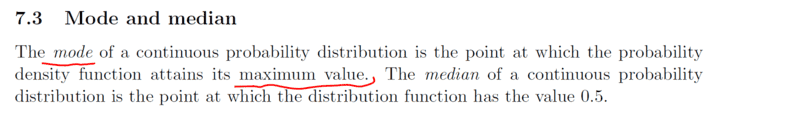# Finding the Mode of a continous distribution

• A
• chwala
In summary, the conversation discusses the mode of a uniform distribution and whether it can have a maximum value. It is clarified that the mode is not always equal to the mean or median, and that for a normal distribution, the mean, mode, and median are all equal. The conversation also briefly touches on the probability of a continuous distribution equaling a specific real number.

#### chwala

Gold Member
TL;DR Summary
I am just going through the literature on Median and mode of continous data. It is quite clear to me on how to get Median i.e from the Function of the probability distribution and also on how to find the Mode i.e which is the gradient of the probability distribution (in essence the probability density function).Attached is my reference on the literature.

My question is; ' are there cases where we may have a continuous distribution that has no Mode value? or is it that the Mode will always be there due to the reason that any given function will have a maximum at some point. Cheers.

What is the mode of a uniform distribution?

Also, a PDF need not have a maximum, for example: $$f : x \mapsto \begin{cases} 0 & x \leq 0 \\ \frac{1}{2\sqrt{x}} & 0 < x < 1 \\ 0 & x \geq 1 \end{cases}$$

pasmith said:
What is the mode of a uniform distribution?

Also, a PDF need not have a maximum, for example: $$f : x \mapsto \begin{cases} 0 & x \leq 0 \\ \frac{1}{2\sqrt{x}} & 0 < x < 1 \\ 0 & x \geq 1 \end{cases}$$
Mode of a uniform distribution ##=0.5## which is also equal to Median = Mean.

pasmith said:
What is the mode of a uniform distribution?

Also, a PDF need not have a maximum, for example: $$f : x \mapsto \begin{cases} 0 & x \leq 0 \\ \frac{1}{2\sqrt{x}} & 0 < x < 1 \\ 0 & x \geq 1 \end{cases}$$
I'll check this later...thanks...

chwala said:
Mode of a uniform distribution ##=0.5## which is also equal to Median = Mean.
Why would the more of a uniform distribution be 0.5? That doesn’t follow from the definition

pasmith said:
What is the mode of a uniform distribution?

Also, a PDF need not have a maximum, for example: $$f : x \mapsto \begin{cases} 0 & x \leq 0 \\ \frac{1}{2\sqrt{x}} & 0 < x < 1 \\ 0 & x \geq 1 \end{cases}$$
Mode would be
Dale said:
Why would the more of a uniform distribution be 0.5? That doesn’t follow from the definition
I got the definition wrong. I thought (it was a general question) and that uniform equates to the Normal distribution. Wrong!

To be clear, the Mode being asked is in reference to post ##2## right...if so then i will check it out.

pasmith said:
What is the mode of a uniform distribution?

Also, a PDF need not have a maximum, for example: $$f : x \mapsto \begin{cases} 0 & x \leq 0 \\ \frac{1}{2\sqrt{x}} & 0 < x < 1 \\ 0 & x \geq 1 \end{cases}$$
...here, the pdf

##f_x =\left[- \dfrac{1}{4\sqrt{x^3}}\right]=\left[-\dfrac{1}{4}+\dfrac{1}{0}\right]##

##x## cannot be defined at ##x=0## thus we would not have a maximum point implying no mode.

•pbuk and Dale
What is the probability that a continuous distribution equals any individual real number?

BWV said:
What is the probability that a continuous distribution equals any individual real number?
? I do not understand your question.

BWV said:
What is the probability that a continuous distribution equals any individual real number?
I think you have confused @chwala: this is not relevant to the definition of the mode. The mode of the normal distribution is 0 because the PDF attains its maximum there.

pbuk said:
I think you have confused @chwala: this is not relevant to the definition of the mode. The mode of the normal distribution is 0 because the PDF attains its maximum there.

True i.e for a Normal distribution Mean = Mode = Median=0

...but I would like to understand that question from @BWV ...

Last edited:
chwala said:
True i.e for a symmetrical Normal distribution Mean = Mode = Median=0

...but I would like to understand that question from @BWV ...
I missed part of your OP where you stated the definition of the mode for a continuous distribution, so not relevant